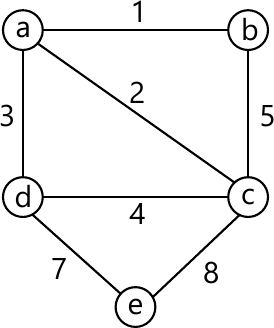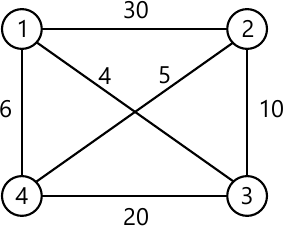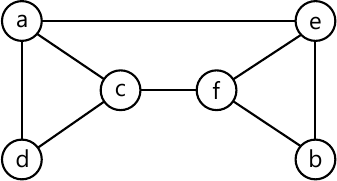MORE IN Design and Analysis of Algorithms
VTU Computer Science (Semester 4)
Design and Analysis of Algorithms
June 2014
Total marks: --
Total time: --
INSTRUCTIONS
(1) Assume appropriate data and state your reasons
(2) Marks are given to the right of every question
(3) Draw neat diagrams wherever necessary

1 (a) Define three asymptotic notations.
6 M
1 (b) Design a recursive algorithm for solving tower of Hanoi problem and give the general plan of analysing that algorithm. Show that the time complexity of tower of Hanoi algorithm is exponential in nature.
8 M
1 (c) With algorithm and suitable example. Explain how the brute force string matching algorithm works. Analyse for its complexity.
6 M

2 (a) Give general divide and conquer recurrence with necessary explanation. Solve the recurrence
T(n)=2T(n/2)+1
T(n)-T(n/2)+n
6 M
2 (b) Explain with suitable example a sorting algorithm that uses divide and conquer technique which divides the problem size by considering position. Give the corresponding algorithms and analyse for time complexity.
8 M
2 (c) Give the problem definition of a defective chessboard? Explain clearly how divide and conquer method can be applied to solve a 4×4 defective chess board problem.
6 M

3 (a) What is job sequencing with deadline problem? Find solution generated by job sequencing problem with deadlines for 7 jobs given profits 3, 5, 20, 18, 1, 6,30 and deadlines 1, 3, 4, 3, 2, 1, 2 respectively.
6 M
3 (b) Define minimum cost spanning tree. Give high level description of Prim's algorithm to find minimum spanning tree and find minimum spanning tree for graph shown in Fig. Q3(b) using Prim's algorithm.8 M
3 (c) What is a knapsack problem? Obtain solution for the knapsack problem using greedy method for n=3, capacity m=20 values 25, 24, 15 and weights 18, 15, 10 respectively.
6 M

4 (a) What is dynamic programming? Explain how would you solve all pair shortest paths problem using dynamic programming.
6 M
4 (b) Give the necessary recurrence relation used to solve O/I knapsack problem using dynamic programming. Apply it to solve the following instance and show the results n=4, m=5 values 12, 10, 20, 15 and weights are 2, 1, 3, 2 respectively.
8 M
4 (c) Solve the following TSP which is represented as graph shown in Fig. 4(c) using dynamic programming.6 M

5 (a) Explain the working of depth-first search algorithm for the graph shown in Fig Q5(a).6 M
5 (b) With pseudo-code, explain how the searching for a pattern BARBER in the given text JIM_SAW_ME_IN_BARBER_SHOP is performed using Horspool's algorithm.
8 M
5 (c) With a suitable example, explain topological sorting.
6 M

6 (a) What is a decision tree? Give decision tree for three element selection sort for arranging three items in ascending order. Give its asymptotic behavior.
6 M
6 (b) What are NP-complete problems and NP-hard problems? Apply four iterations of Newton's method to compare ?2 and estimate the absolute and relative errors of the computations.
8 M
6 (c) What do you mean by lower bound algorithm? What are the advantages of finding the lower bound and give different methods of obtaining the lower bound?
6 M

7 (a) With necessary state space diagram, explain the solving of four-queen's problem by backtracking.
10 M
7 (b) For the given n×n cost matrix C for a job assignment problem find optimal solution using branch and bound method. Give complete state-tree for the instance of assignment problem solved with best first branch and bound algorithm. $C= \begin{matrix} \begin{matrix} j1 &j2 &j3 &j4 \ \ \ \ \ \ \ \ \ \ \ \ \ \end{matrix}\\ \begin{bmatrix}9 &2 &7 &8 \\6 &4 &3 &7 \\5 &8 &1 &8 \\7 &6 &9 &4 \end{bmatrix} \begin{matrix}person\ a\\person \ b \\person \ c \\person \ d \\ \end{matrix} \end{matrix}$
10 M

8 (a) Explain different types of computation models.
10 M
8 (b) For given input 5, 12, 8, 6, 3, 9, 11, 12, 1, 5, 6, 7, 10, 4, 3, 5 to the prefix computation problem and ? stands for addition solve problem using work optimal algorithm.
10 M

More question papers from Design and Analysis of Algorithms